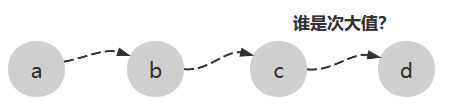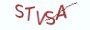# 1.3.1 算法思路# 1.3.2 算法实现

```# __author__ = 薯条老师
import random
x = random.randint(0, 1000)
y = random.randint(0, 1000)
z = random.randint(0, 1000)
k = random.randint(0, 1000)

# 计算三个中的最大值
max_ = x if x > y else y
if z > max_:
max_ = z

# 计算三个中的最小值
min_ = x if x < y else y
if z < min_:
min_ = z

# 将三个变量相加再减去最大值和最小值，结果一定是中值
median = x+y+z-max_-min_

# 假设三个中的中值为次大值
max_k2 = median

if k > max_:
# 比三个中的最大值max_要大，次大值就是max_
max_k2 = max_
elif k > median:
# 比max_小但比median大，那么次大值就是k
max_k2 = k

print("次大值:{}".format(max_k2))```

# 1.3.3 最具实力的小班培训

`(1) Python后端工程师高薪就业班，月薪11K-18K，免费领取课程大纲(2) Python爬虫工程师高薪就业班，年薪十五万，免费领取课程大纲(3) Java后端开发工程师高薪就业班，月薪11K-20K, 免费领取课程大纲(4) Python大数据分析，量化投资就业班，月薪12K-25K,免费领取课程大纲`## 注册账号